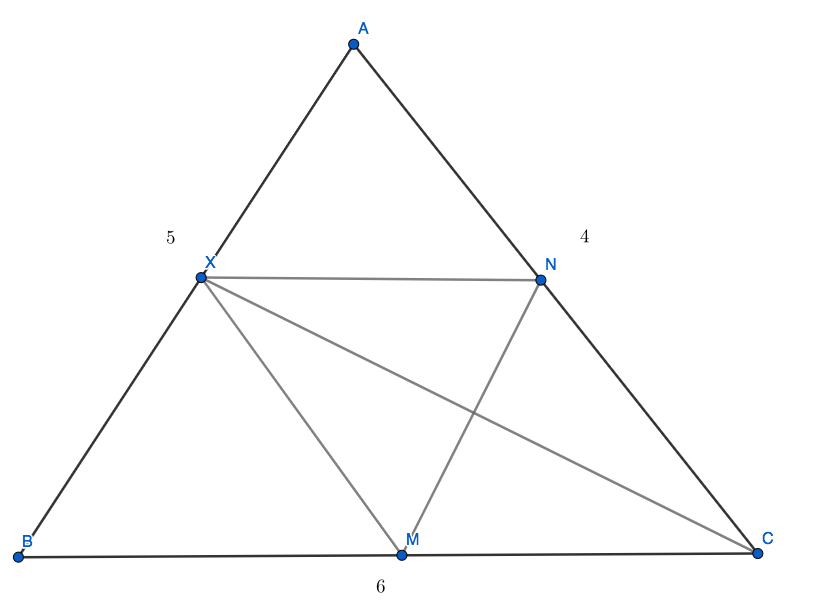# Harvard MIT MT

Geometry Level 4Let $ABC$ be a triangle with $AB = 5, AC = 4, BC = 6$. The angle bisector of $C$ intersects side $AB$ at $X$. Points $M$ and $N$ are drawn on sides $BC$ and $AC$, respectively, such that $\overline { XM } \parallel \overline { AC }$ and $\overline { XN } \parallel \overline { BC }$. Compute the length $MN$.

×